## Null Space

A homogeneous linear system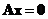can be thought as a linear transformation of a vector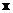in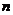dimensional space into zero vector in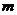dimensional space.

The solutionof a homogeneous system of equationsis called the null space of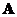and the dimension of the null space is called the nullity of.

The dimension of the range and the null space of a matrix are related through fundamental relationship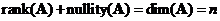. Whereis the number of original unknowns.